# Lecture 11: Coordinate Transformations, Recursion & Self-similarity I

## Annoucements

Assignment 06 Due Friday MONDAY!!!

• tester later this week

## Outline

1. Coordinates
2. Coordinate Transformations
3. Koch Curve Activity
4. SVG Groups and Coordinates

## Motivation: Self-Similarity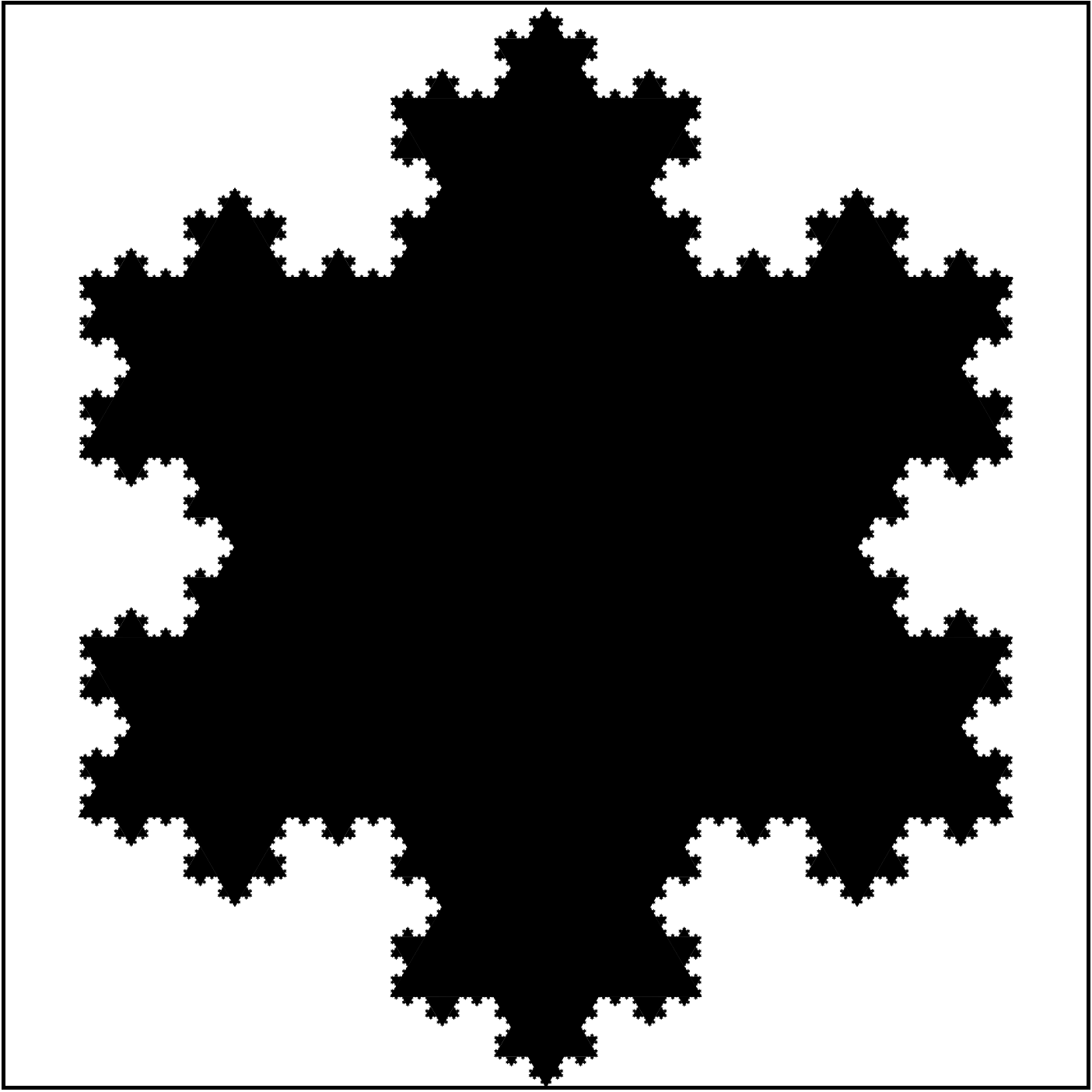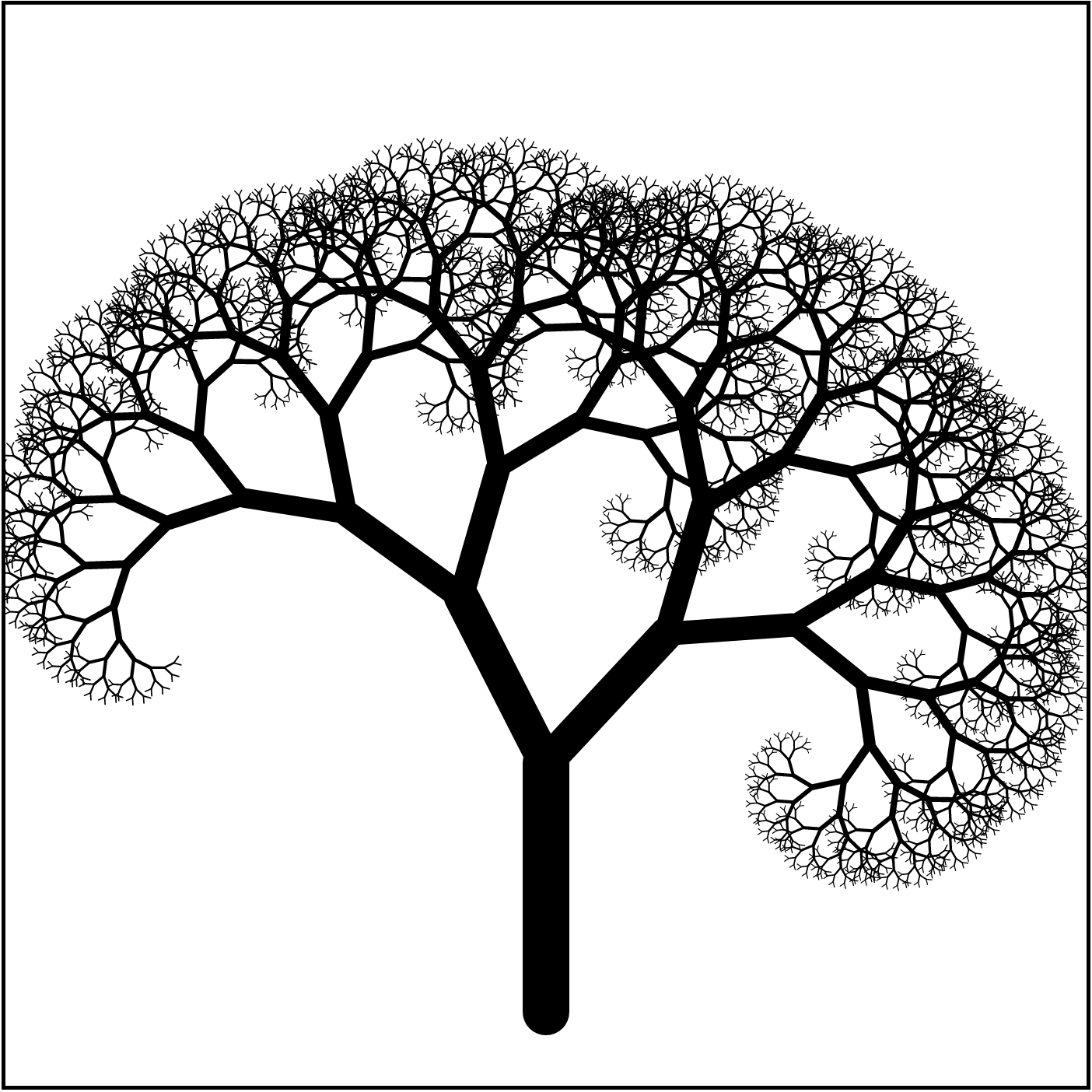## Goal

Generate self-similar graphical content

Homework 07: draw self-similar images

## Coordinates

Cartessian Coordinates:

• associate each point in the plane with a pair of numbers: $A = (x, y)$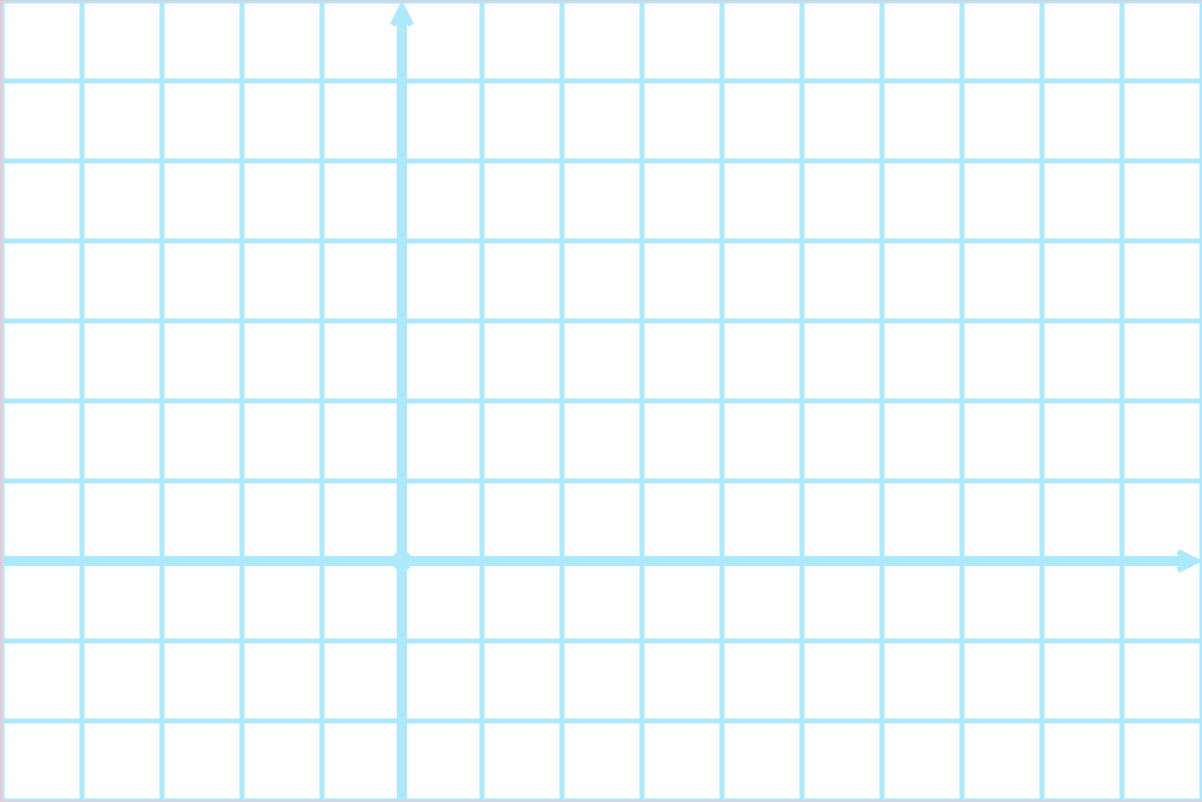## Screen vs Standard Coordinates

Screen coordinates:

• origin is upper left corner
• $x$ increases in right direction
• $y$ increases in downward direction

Standard coordinates:

• origin is somewhere
• depends on region we want to depict
• $x$ in right direction
• $y$ increases in upward direction

Today: use standard coordinates!

## Points and Vectors

• a point is a location in the plane
• specified by a pair of numbers: coordinates
• a vectors is a displacement between points
• magnitude + direction
• arrows
• specified by a pair of numbers: components

## Points and Vectors, Illustrated## Vector Operations

Vectors can be manipulated with algebraic operations:

• $(u_1, u_2) + (v_1, v_2) = (u_1 + v_1, u_2 + v_2)$
2. scalar multiplication:
• $c (u_1, u_2) = (c u_1, c u_2)$

Coordinates can be interpreted as vectors:

• associate the point $A = (x, y)$ with the vector $(x, y)$

## Vector Operations Illustrated## Basic Coordinate Transformations

SVG supports transformation of elements (shapes, groups, etc)

• translate(tx, ty): take each vector $(a, b)$ and move it to $(a + t_x, b + t_y)$
• scale(s): take each vector $(a, b)$ and move it to $(s \cdot a, s \cdot b)$
• rotation(d): rotate each vector by $d$ degrees in the counter clockwise direction around the origin

For example:

<rect width="20" height="20" transform="translate(30, 40)"/>


## Translation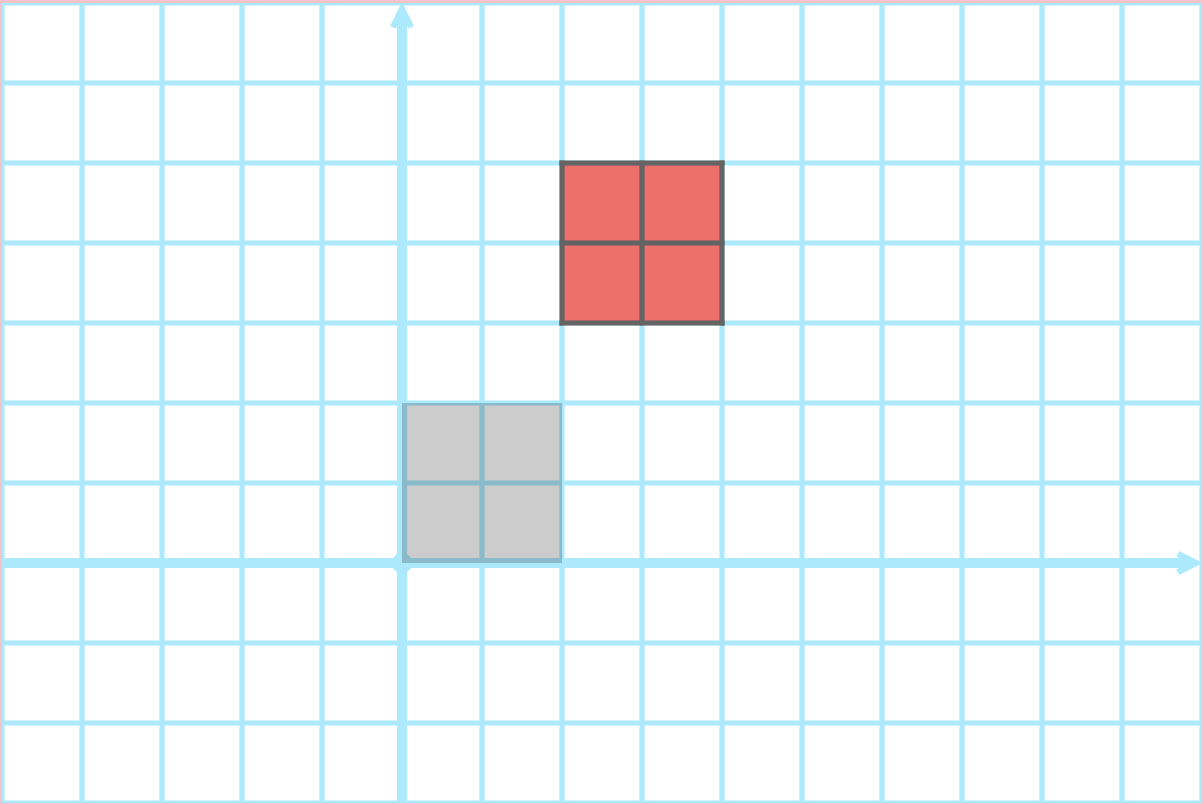## Scale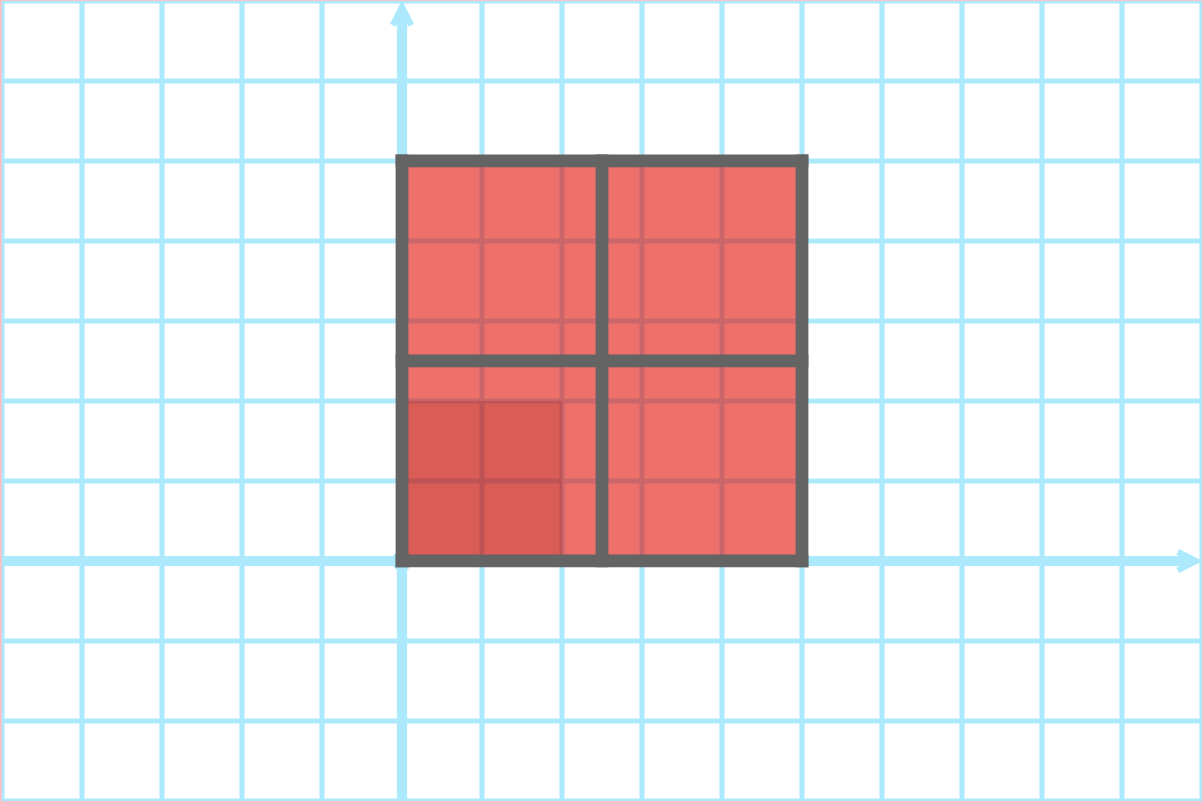## Rotation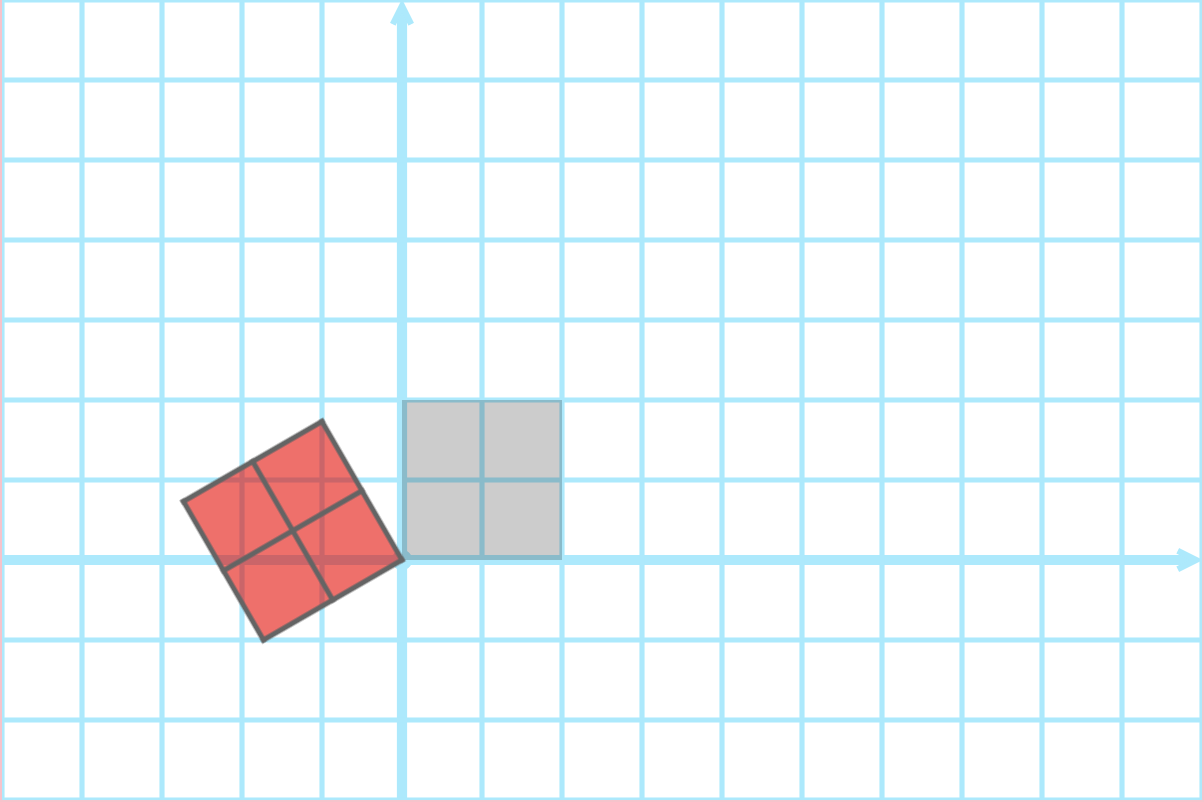## Composing Transformations

Transformations can be composed:

• perform one transformation, then another, …
• transformations are applied
• in order “left to right”
• relative to previously transformations

## Translation then Rotation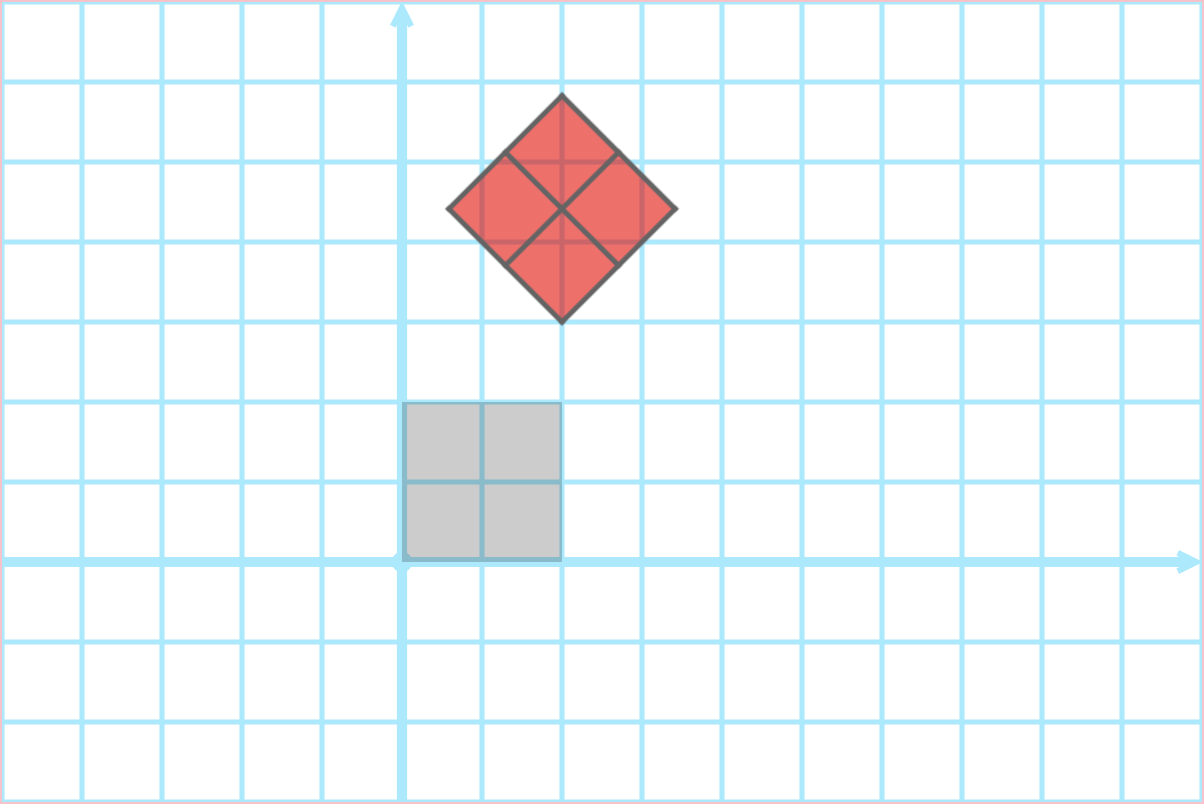## Rotation then Translation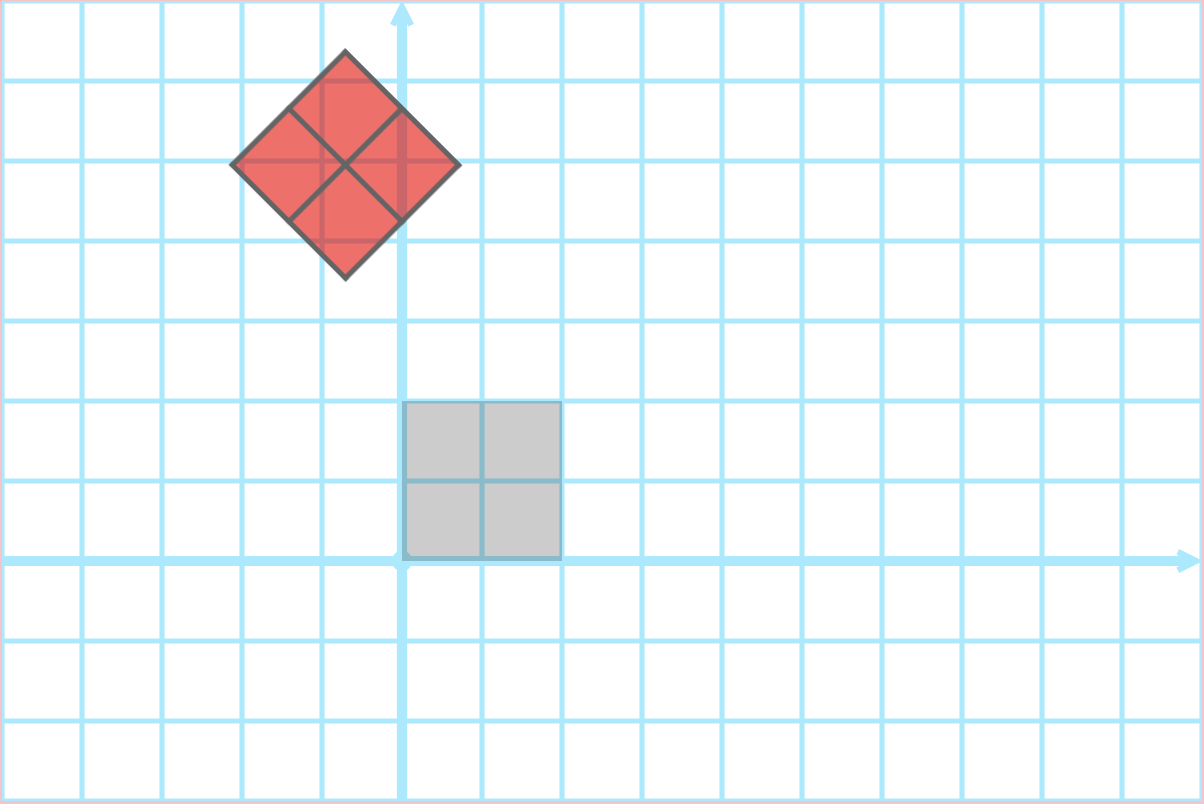## Demo

• lec11-coordinate-transformations.zip

## translate(-20, 10) scale(2)?## scale(2) translate(-20, 10)?## More Generally

A broad class of transformations are defined by:

1. how they transform the first standard basis vector $e_1 = (1, 0)$
2. how they transform the second standard basis vector $e_2 = (0, 1)$
3. how they transform the origin $(0, 0)$

## Affine Transformations

Suppose a transformation maps:

• vector $(1, 0)$ to $(a, b)$
• vector $(0, 1)$ to $(c, d)$
• point $(0, 0)$ to $(e, f)$

To apply transformation to $(x, y)$:

1. write $(x, y) = x (1, 0) + y (0, 1)$
2. apply transformation to $(1, 0)$ and $(0, 1)$
3. get resulting value: $x (a, b) + y (c, d) = (ax + cy, bx + dy)$
4. add $(e, f)$ to result:

$(ax + cy + e, bx + dy + f)$

This is an affine transformation

## matrix Transformations

In SVG you can perform an affine transformation

• vector $(1, 0)$ to $(a, b)$
• vector $(0, 1)$ to $(c, d)$
• point $(0, 0)$ to $(e, f)$

with

transform=matrix(a, b, c, d, e, f)


matrix transforms include all scale, translate, rotate transforms, and more!

## Questions

1. What is the matrix equivalent of translate(20, 30)?

2. What is the matrix equivalent of scale(2)?

3. What is the matrix equivalent of rotate(90)?

## Question

What is the matrix of this transformation?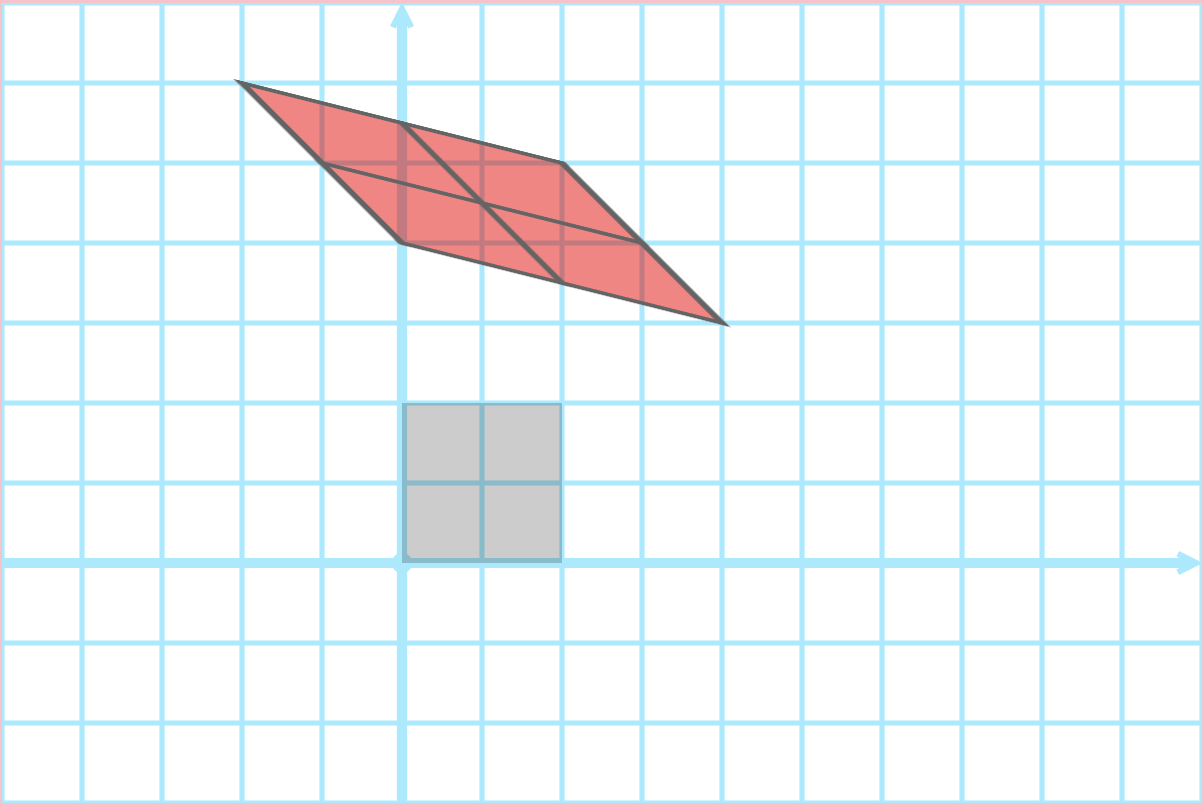## Self-Similarity via Transformations## Example: Koch Curve I

How did we make the snowflake fractal?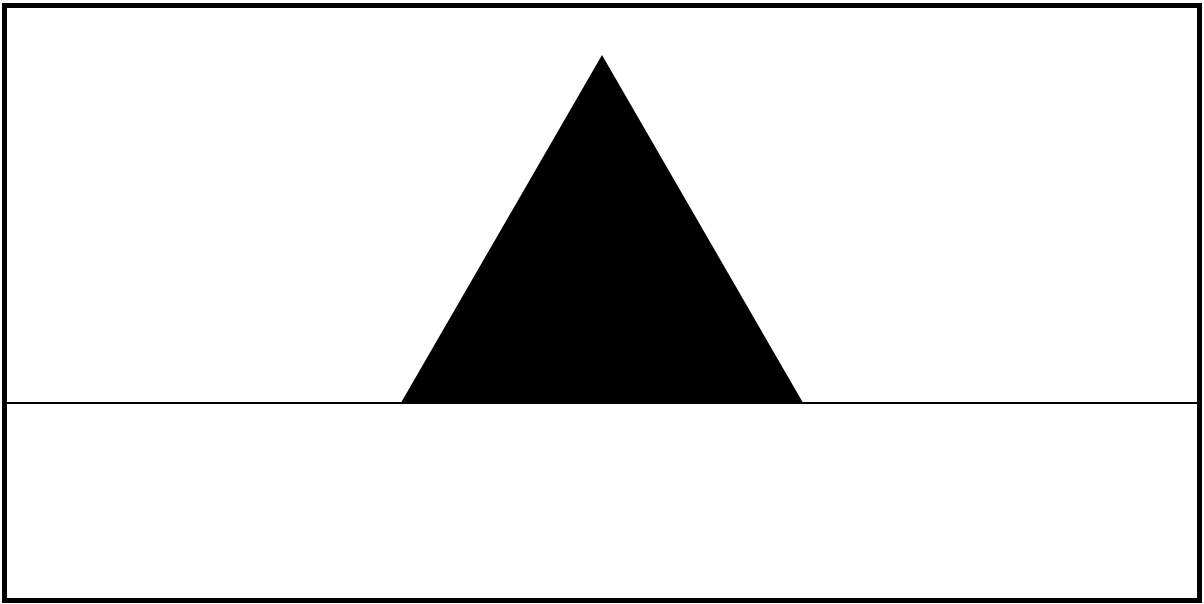Step 1: define a basic shape

## Example: Koch Curve II

How did we make the snowflake fractal?Step 2: define sub-shapes for basic shape

## Example: Koch Curve III

How did we make the snowflake fractal?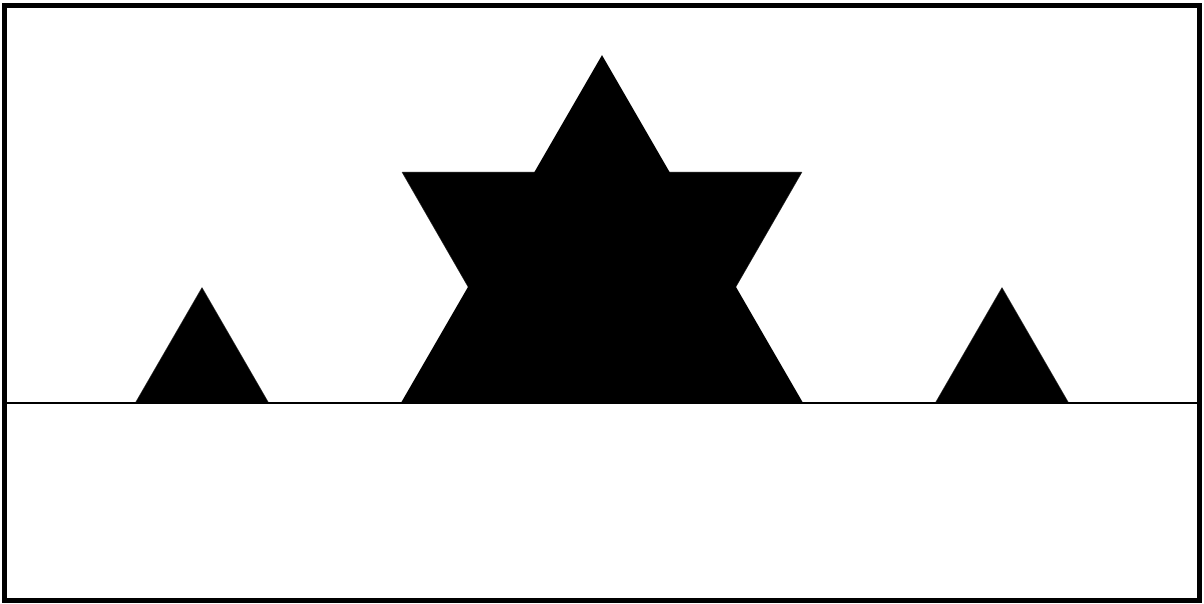Step 3: recurse

## Example: Koch Curve IV

How did we make the snowflake fractal?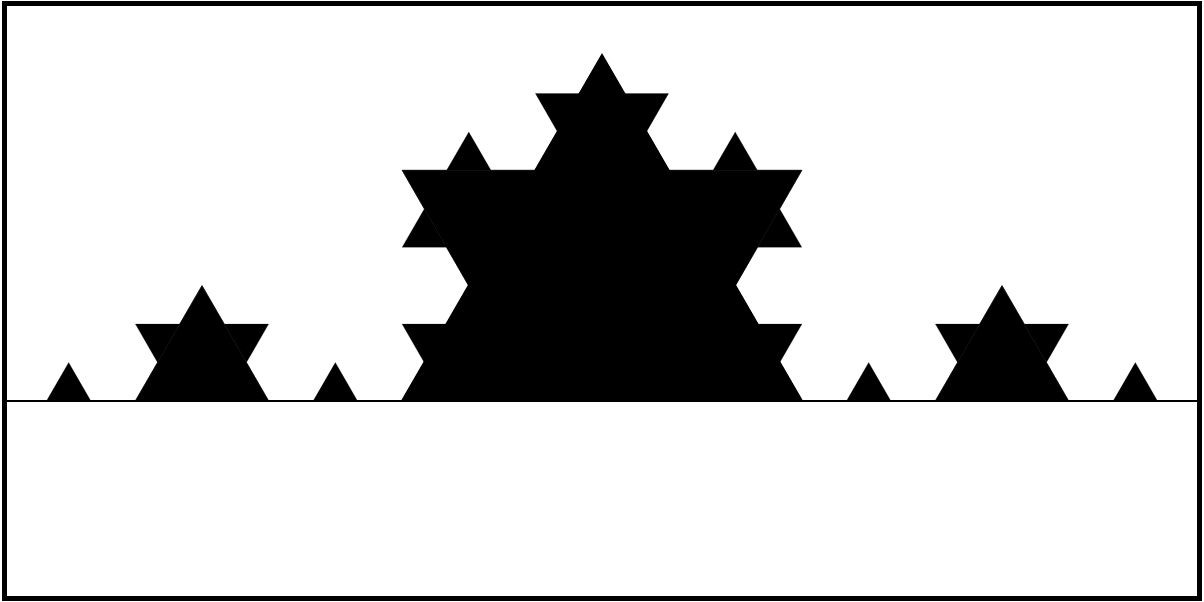Step 3: recurse

## ObservationEach iteration draws a bunch of transformed copies of the original shape

## Repetition and Transformation in SVG

1. Define the basic shape in a <defs> element

 <defs>
<rect id="my-rect" width="20" height="20"/>
</defs>

2. Draw basic shape with <use>, apply transform to transform the element

 <use href="#my-rect" transform="translate(20, 30)"/>


Now can re-use my-rect over and over again with different transformations

• of course, this can (should?) all be done with JavaScript

## Activity

Draw two iterations of the Koch curve!

• lec11-koch-step.zip

## Next TimeMake things easier!

• compose transformations by nesting group (<g>) elements
• program drawing recursively